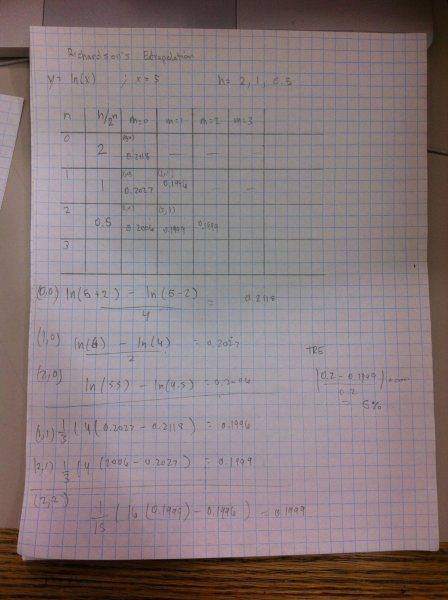# Richardson Extrapolation with 3 steps?

## Homework Statement

[/B]
use richardson extrapolation to estimate the first derivative y=ln(x), x=5 using steps of 2, 1, 0.5. Four decimal points. obtain true relative error for the last estimate and comment on its value.

[/B]
deriv ln(x)=1/x

## The Attempt at a Solution

I know for step sizes of h and h/2 the formula is...

f'(x)=1/3 [ 4*[f(x+h/2)/h-f(x-h/2)/h] - f(x+h)/h-f(x-h)/h] + O(h^4)

but if im using steps h, h/2, h/4 the formula should be different, right?

or do you run the first step to find an approximation, then run it again using the next step?

Any help is appreciated!

Thanks

STEMucator
Homework Helper
To obtain the true error, you're going to need to compute ##f'(5) = \frac{1}{5} = 0.2##.

As for estimating the derivative itself, you must choose to use forward, backward or centered difference formulas. For example, if we wanted an estimate with error proportional to ##O(h^2)##, we could use the centered formula:

$$f'(x_i) = \frac{f(x_{i+1}) - f(x_{i-1})}{2h}$$

With ##x_i = 5##, ##x_{i+1} = 6## and ##x_{i-1} = 4##, the equation takes the form:

$$f'(5) = \frac{f(6) - f(4)}{2h} = \frac{ln(6) - ln(4)}{2}$$

This equation has step size ##h=1##. You can compute the true error for each result for each step size.

Now you will have three estimates for the derivative, presuming you evaluated the centered difference equation for all step sizes. All of these estimates have error proportional to ##O(h^2)##. Using Richardson extrapolation, a high accuracy result can be determined from these estimates. First an estimate with error proportional to ##O(h^4)## can be determined from:

$$\frac{4}{3} D(h_2) - \frac{1}{3} D(h_1)$$

This only works if ##h_2 = \frac{h_1}{2}##.

Then a really accurate estimate with error proportional to ##O(h^6)## can be determined from:

$$\frac{16}{15} D(h_2) - \frac{1}{15} D(h_1)$$

•dmoney123
Thank you!

So you would run the central difference formula with 2, 1, 0.5.

then for

4/3D(h2)−1/3D(h1)

we would use f(2) and f(1) , and then again with f(1), f(0.5)?

Maybe what confuses me is when they say "calculate the TRE of the last estimate", its just the second of two estimates calculated using

4/3D(h2)−1/3D(h1)

Is it possible to derive a formula that has 3 terms that includes h_3, h_2, h_1 where h_1=h_2/2=h_3/4

STEMucator
Homework Helper
So you would run the central difference formula with 2, 1, 0.5.

Yes, but you need to choose different ##x_i, x_{i+1}, x_{i-1}## for each step size. Notice the values of these variables in the prior post with step size ##h = 1##.

Maybe what confuses me is when they say "calculate the TRE of the last estimate", its just the second of two estimates calculated using

To compute the true relative error for each result, simply use:

$$E_T = \frac{|\text{true result - calculated result}|}{\text{true result}} \times 100 \% = \frac{|0.2 - \text{calculated result}|}{0.2} \times 100 \%$$

You can compute one for each calculated result, but the question only wants to you comment on a particular one.

then for

Zondrina said:
4/3D(h2)−1/3D(h1)

we would use f(2) and f(1) , and then again with f(1), f(0.5)?

No, think about what each ##D(h_i)## represents in terms of the estimates you have calculated. You should also take notice of the spacing between each step size ##h_i## and how it relates to ##h_2 = \frac{h_1}{2}##.

Is it possible to derive a formula that has 3 terms that includes h_3, h_2, h_1 where h_1=h_2/2=h_3/4

I don't think so. The point of Richardson Extrapolation is to recursively compute better derivative/integral estimates. There are actual formulas for this, namely:

$$\frac{4}{3} D(h_2) - \frac{1}{3} D(h_1)$$

$$\frac{16}{15} D(h_2) - \frac{1}{15} D(h_1)$$

There is an actual pattern here, and the next term would be ##\frac{64}{63} D(h_2) - \frac{1}{63} D(h_1)##.

The first equation above takes two estimates with error proportional to ##O(h^2)## and satisfies ##h_2 = \frac{h_1}{2}##; it allows you to compute an estimate with error proportional to ##O(h^4)##. So the first equation looks something like this for the problem at hand:

$$\frac{4}{3} D(h_2) - \frac{1}{3} D(h_1) = \frac{4}{3} D(\frac{h_1}{2}) - \frac{1}{3} D(h_1) = \frac{4}{3} D(1) - \frac{1}{3} D(2)$$

•dmoney123i think i got it.
thank you very much zondrina!

STEMucator
Homework Helper
Your process looks okay, but i think some of the numbers might be off.

I get 0.21735 for the ##O(h^4)## estimate and 0.20053 for the ##O(h^6)## estimate, which as you may notice is very close to the real value. I think you may have forgotten some brackets or something.